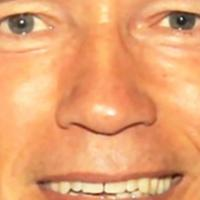# Aligning face images

Some people have asked me how I've aligned the face images in my articles. Giving people my ImageMagick hack is embarrassing for me, so I've decided to rewrite it into a Python script. You don't need to copy and paste it, as the script comes with the source folder of my Guide on Face Recognition: crop_face.py.

The code is really easy to use. To scale, rotate and crop the face image you just need to call `CropFace(image, eye_left, eye_right, offset_pct, dest_sz)`, where:

• `eye_left` is the position of the left eye
• `eye_right` is the position of the right eye
• `offset_pct` is the percent of the image you want to keep next to the eyes (horizontal, vertical direction)
• `dest_sz` is the size of the output image

If you are using the same `offset_pct` and `dest_sz` for your images, they are all aligned at the eyes.

## example

Imagine we are given this photo of Arnold Schwarzenegger, which is under a Public Domain license. The `(x,y)`-position of the eyes are approximately `(252,364)` for the left and `(420,366)` for the right eye. Now you only need to define the horizontal offset, vertical offset and the size your scaled, rotated & cropped face should have. Here are some examples:

Configuration Cropped, Scaled, Rotated Face
0.1 (10%), 0.1 (10%), (200,200)0.2 (20%), 0.2 (20%), (200,200)0.3 (30%), 0.3 (30%), (200,200)0.2 (20%), 0.2 (20%), (70,70)## crop_face.py

```#!/usr/bin/env python
# Software License Agreement (BSD License)
#
# Copyright (c) 2012, Philipp Wagner
# All rights reserved.
#
# Redistribution and use in source and binary forms, with or without
# modification, are permitted provided that the following conditions
# are met:
#
#  * Redistributions of source code must retain the above copyright
#    notice, this list of conditions and the following disclaimer.
#  * Redistributions in binary form must reproduce the above
#    copyright notice, this list of conditions and the following
#    disclaimer in the documentation and/or other materials provided
#    with the distribution.
#  * Neither the name of the author(s) nor the names of its
#    contributors may be used to endorse or promote products derived
#    from this software without specific prior written permission.
#
# THIS SOFTWARE IS PROVIDED BY THE COPYRIGHT HOLDERS AND CONTRIBUTORS
# "AS IS" AND ANY EXPRESS OR IMPLIED WARRANTIES, INCLUDING, BUT NOT
# LIMITED TO, THE IMPLIED WARRANTIES OF MERCHANTABILITY AND FITNESS
# FOR A PARTICULAR PURPOSE ARE DISCLAIMED. IN NO EVENT SHALL THE
# COPYRIGHT OWNER OR CONTRIBUTORS BE LIABLE FOR ANY DIRECT, INDIRECT,
# INCIDENTAL, SPECIAL, EXEMPLARY, OR CONSEQUENTIAL DAMAGES (INCLUDING,
# BUT NOT LIMITED TO, PROCUREMENT OF SUBSTITUTE GOODS OR SERVICES;
# LOSS OF USE, DATA, OR PROFITS; OR BUSINESS INTERRUPTION) HOWEVER
# CAUSED AND ON ANY THEORY OF LIABILITY, WHETHER IN CONTRACT, STRICT
# LIABILITY, OR TORT (INCLUDING NEGLIGENCE OR OTHERWISE) ARISING IN
# ANY WAY OUT OF THE USE OF THIS SOFTWARE, EVEN IF ADVISED OF THE
# POSSIBILITY OF SUCH DAMAGE.

import sys, math, Image

def Distance(p1,p2):
dx = p2 - p1
dy = p2 - p1
return math.sqrt(dx*dx+dy*dy)

def ScaleRotateTranslate(image, angle, center = None, new_center = None, scale = None, resample=Image.BICUBIC):
if (scale is None) and (center is None):
return image.rotate(angle=angle, resample=resample)
nx,ny = x,y = center
sx=sy=1.0
if new_center:
(nx,ny) = new_center
if scale:
(sx,sy) = (scale, scale)
cosine = math.cos(angle)
sine = math.sin(angle)
a = cosine/sx
b = sine/sx
c = x-nx*a-ny*b
d = -sine/sy
e = cosine/sy
f = y-nx*d-ny*e
return image.transform(image.size, Image.AFFINE, (a,b,c,d,e,f), resample=resample)

def CropFace(image, eye_left=(0,0), eye_right=(0,0), offset_pct=(0.2,0.2), dest_sz = (70,70)):
# calculate offsets in original image
offset_h = math.floor(float(offset_pct)*dest_sz)
offset_v = math.floor(float(offset_pct)*dest_sz)
# get the direction
eye_direction = (eye_right - eye_left, eye_right - eye_left)
# calc rotation angle in radians
rotation = -math.atan2(float(eye_direction),float(eye_direction))
# distance between them
dist = Distance(eye_left, eye_right)
# calculate the reference eye-width
reference = dest_sz - 2.0*offset_h
# scale factor
scale = float(dist)/float(reference)
# rotate original around the left eye
image = ScaleRotateTranslate(image, center=eye_left, angle=rotation)
# crop the rotated image
crop_xy = (eye_left - scale*offset_h, eye_left - scale*offset_v)
crop_size = (dest_sz*scale, dest_sz*scale)
image = image.crop((int(crop_xy), int(crop_xy), int(crop_xy+crop_size), int(crop_xy+crop_size)))
# resize it
image = image.resize(dest_sz, Image.ANTIALIAS)
return image

if __name__ == "__main__":
image =  Image.open("arnie.jpg")
CropFace(image, eye_left=(252,364), eye_right=(420,366), offset_pct=(0.1,0.1), dest_sz=(200,200)).save("arnie_10_10_200_200.jpg")
CropFace(image, eye_left=(252,364), eye_right=(420,366), offset_pct=(0.2,0.2), dest_sz=(200,200)).save("arnie_20_20_200_200.jpg")
CropFace(image, eye_left=(252,364), eye_right=(420,366), offset_pct=(0.3,0.3), dest_sz=(200,200)).save("arnie_30_30_200_200.jpg")
CropFace(image, eye_left=(252,364), eye_right=(420,366), offset_pct=(0.2,0.2)).save("arnie_20_20_70_70.jpg")
```